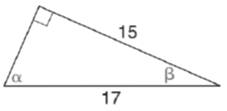Chapter 11.3, Problem 2EElementary Geometry For College St...

7th Edition
Alexander + 2 others
ISBN: 9781337614085

Solutions

Chapter
SectionElementary Geometry For College St...

7th Edition
Alexander + 2 others
ISBN: 9781337614085
Textbook Problem

In Exercises 1 to 4, find tan α and tan β for each triangle.To determine

To find:

The values of tanα and tanβ for the triangle.

Explanation

Given:

The triangle is shown below.

Definition used:

In a right triangle, the tangent ratio for an acute angle is the ratio oppositeadjacent.

Calculation:

In a right triangle, (hypotenuse)2=(opposite)2+(adjacent)2

Let x be the unknown side in the given figure.

(17)2=x2+152289=x2

Still sussing out bartleby?

Check out a sample textbook solution.

See a sample solution

The Solution to Your Study Problems

Bartleby provides explanations to thousands of textbook problems written by our experts, many with advanced degrees!

Get Started

Define the alpha level and the critical region for a hypothesis test.

Statistics for The Behavioral Sciences (MindTap Course List)

Change 325 to an improper fraction.

Elementary Technical Mathematics

In Exercises 1-6, simplify the expression. (3x)+(x+3)3

Calculus: An Applied Approach (MindTap Course List)

Divide the following fractions and reduce to lowest terms. 28.

Contemporary Mathematics for Business & Consumers

In Exercises 724, compute the indicated products. 

Finite Mathematics for the Managerial, Life, and Social Sciences

Polar coordinates of the point with rectangular coordinates (5, 5) are: (25, 0)

Study Guide for Stewart's Single Variable Calculus: Early Transcendentals, 8th